# What is the difference between the `Orientation` and `Rotation` propertry

## What I tried

Could not find any information regarding this question via Google Chrome Search Engine or the Site Search feature.

I tried looking at the Wiki documentation but could not find out how either are derived and why there is a difference in `Orientation` and `Rotation` in some cases for parts facing the same direction.

The only information I could scrape is how the property is applied (Orientation wiki page)

The Orientation property describes the part’s rotation in degrees around the X, Y and Z axes using a Vector3. The rotations are applied in Y → X → Z order. This differs from proper Euler angles, and is instead Tait–Bryan angles which describe yaw, pitch and roll .

I didn’t really understand this however and attempting to read the Wikipedia article just confused me more.

## The Problem

I don’t know the difference between the `Orientation` and `Rotation` property. They appear to be the same in some cases, but in others they’re totally opposite.
I tested this code block twice and got different results:
`local part = workspace.Part print(part.Orientation) warn(part.Rotation)`

### Case 1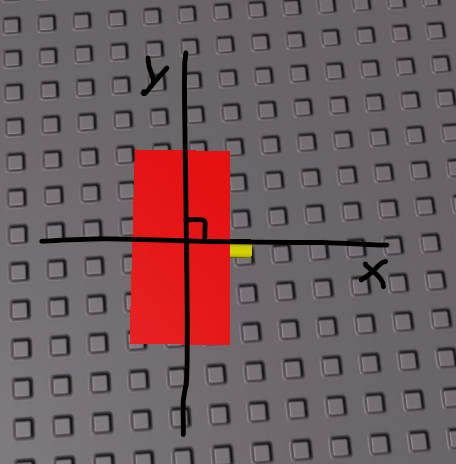Which produced the results:

0, -95, 0
-180, -85, -180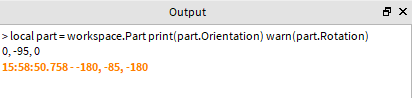### Case 2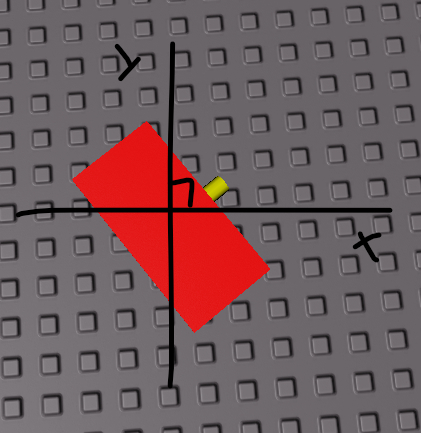Which produced the results:

0, -55, 0
0, -55, 0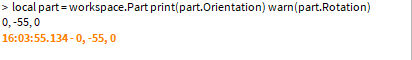I am confused as of why there is a difference in the values, and why it only happens in some cases? I noticed the rotation property appears to be the rotation minus 180 in most cases.

1 Like

The Orientation and Rotation properties use a system known as Euler angles to define the rotation of the part in 3D space. There are three components, known in Roblox as X, Y and Z, which correspond to the angles of pitch, yaw and roll respectively.

The order in which you perform each one of those three rotations is important; applying them in a different order will produce a different result. This is what is meant when the docs say that Rotation is applied in XYZ order and Orientation applied in YXZ order.

You can think of it in terms of constructing the part’s CFrame. Rotation would look like this;

``````part.CFrame = CFrame.new(position)
* CFrame.Angles(pitch, 0  , 0   ) --X
* CFrame.Angles(0    , yaw, 0   ) --Y
* CFrame.Angles(0    , 0  , roll) --Z
``````

Whereas Orientation would look like this;

``````part.CFrame = CFrame.new(position)
* CFrame.Angles(0    , yaw, 0   ) --Y
* CFrame.Angles(pitch, 0  , 0   ) --X
* CFrame.Angles(0    , 0  , roll) --Z
``````

Notice how the order the angles are applied changes the final CFrame, as CFrame multiplication is non-associative (or in regular terms, cframe1 * cframe2 is not the same as cframe2 * cframe1).

8 Likes

If the Rotation is applied in `XYZ` and Orientation is applied in `YXZ` shouldn’t I expect to see a different output?

Why does `blue.Rotation = Vector3.new(12, 50, 179)` and `red.Orientation = Vector3.new(12, 50, 180)` result in the same output.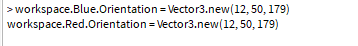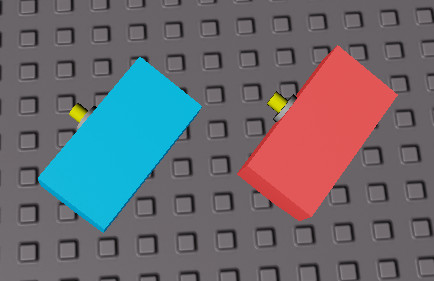2 Likes

There’s not always a significant difference between the two systems in certain cases like these; a more extreme example might be the identity rotation (0, 0, 0), which in both systems represents no rotation/orientation. Where the distinction matters is when you care about the axes of rotation which your angles represent; Euler angles rotate the axes of rotation as you apply each rotation.

2 Likes

I’m confused. Why is there no significant difference in the way I apply my rotation? Certainly changing an object’s rotation by -30 Z degrees is different than -30 Y degrees? Or is something different implied when rotation in `XYZ` vs `YXZ`

Also what do you mean by this:

Alright, I’ll make a quick visual; there used to be a good GIF showing it on the old wiki that I can’t find anymore.

1 Like

Let’s take a regular part with no initial rotation. Here, I’m highlighting the axes of rotation (red = X, green = Y, blue = Z);

In the Euler angles system that Roblox uses, the axes of rotation will rotate along with the part. So, if we apply a 45 degree rotation on the X axis, notice that the Y and Z axes are also rotated:

If we then apply a 90 degree rotation on the Y axis, the axes will be rotated again;

Now, let’s try that again, but we’ll do the Y axis first. So here’s our starting part again:

We rotate 90 degrees around the Y axis:

Then, 45 degrees around the X axis:

If we compare the two side by side, you’ll notice that, due to the axes of rotation being rotated, the two final rotations are not the same:

This is why there is a difference between Rotation (X axis first, Y axis second, Z axis last) and Orientation (Y axis first, X axis second, Z axis last).

With that being said, you can still sometimes get rotations that end up being the same if you use certain angles; for example, let’s rotate 180 degrees on the X and Y axes. On the left I’ve done the X rotation first, and on the right the Y rotation first:

I wouldn’t rely on this behaviour, however, as it’s merely a coincidence if/when it happens. It’s always better to think about the order of your rotations when choosing whether to use Rotation or Orientation.

21 Likes

Thank you so much for the help; the visual representation helped majorly.

2 Likes

because in the picture you make both Orientation. that’s why it’s the same, just saying if it still confuses u